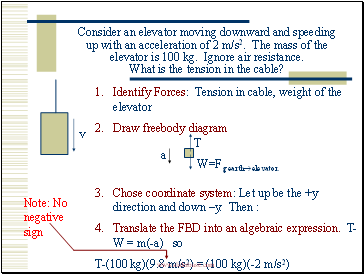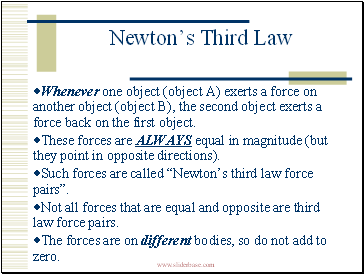# Newton's laws of motionPage 2

#### WATCH ALL SLIDES

Slide 9Consider an elevator moving downward and speeding up with an acceleration of 2 m/s2. The mass of the elevator is 100 kg. Ignore air resistance. What is the tension in the cable?

v

Identify Forces: Tension in cable, weight of the elevator

Draw freebody diagram

Chose coordinate system: Let up be the +y direction and down –y. Then :

Translate the FBD into an algebraic expression. T-W = m(-a) so

T-(100 kg)(9.8 m/s2) = (100 kg)(-2 m/s2)

Note: No negative sign

Slide 10## Newton’s Third Law

Whenever one object (object A) exerts a force on another object (object B), the second object exerts a force back on the first object.

These forces are ALWAYS equal in magnitude (but they point in opposite directions).

Such forces are called “Newton’s third law force pairs”.

Not all forces that are equal and opposite are third law force pairs.

The forces are on different bodies, so do not add to zero.

Go to page:
1  2